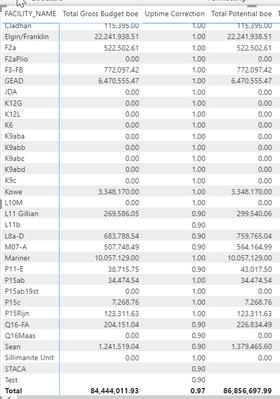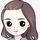cancel
Showing results for
Did you mean:Helper I

## Totals of divde function

Hi,

I am trying to sum up after a division calculation based on the "facility_id" filter.

I have seen some solutions using HASONEFILTER and it feels like I'm getting there, but still not quite.

At the moment I'm trying to assess the potential on a facility level and this works.

But then when summing it up, the total is wrong as it divides on row level.

This is my data table which is correct on a row level:My measures are:

``````Total Potential boe = [Total Gross Budget boe]/[Uptime Correction]

Uptime Correction = CALCULATE(AVERAGE(Facility_Lookup[_Uptime]),Facility_Lookup[Facility_ID])

Total Gross Budget boe = [100% Budget Gas boe]+[100% Budget Oil]

100% Budget Oil = CALCULATE(sum('flow_data_forecast'[FL_DATA_NET_VOL]), 'flow_data_forecast'[FORECAST_ID]=1,flowID_Lookup[_Oil Sales Daily Data]=1)
100% Budget Gas boe = [100% Budget Gas Nm3]*0.0062897
100% Budget Gas Nm3 = CALCULATE(sum('flow_data_forecast'[FL_DATA_NET_VOL]), 'flow_data_forecast'[FORECAST_ID]=1,flowID_Lookup[_Gas Sales Daily Data]=1)``````

So any help is appreciated.

Regards,

Gerlof

1 ACCEPTED SOLUTIONCommunity Support

When to Use SUM() vs SUMX()

Best Regards

Rena

Community Support Team _ Rena
If this post helps, then please consider Accept it as the solution to help the other members find it more quickly.
6 REPLIES 6Super User

@GerlofVisser , Somehow I am not able to find the sumx calculation

You need to

sumx(Values(Table[facility_id]), <Calculation>)Helper I

Hi @amitchandak ,

Thanks, that will help with my further queries, but you are right, this was no sumx issue.

I noticed there is also an error with summing up my "Total Potential", the actual sum of this column 84,771,290.7 (after export to excel).Community Support

Please try to create a new measure and update the formula of measure [Total Potential boe] as below under the premise that the total value of measure [Total Gross Budget boe] is correct.

1. Create a new measure [Measure]

``Measure =SUMX ( VALUES ( 'Facility_Lookup'[FACILITY_NAME] ), [Uptime Correction] )``

2. Update the formula of measure [Total Potential boe]

``Total Potential boe =[Total Gross Budget boe] / [Measure]``

In addition, you can try the methods in the following links to handle it.

Totals Incorrect

Measure Totals, The Final Word

Why Your Total Is Incorrect In Power BI

If all of them are not working, please provide some sample data and the correct values(exclude sensitive data) in form of table. It is better if you can provide your sample pbix file. Thank you.

Best Regards

Rena

Community Support Team _ Rena
If this post helps, then please consider Accept it as the solution to help the other members find it more quickly.Helper I

I have mentioned to get the correct values, by using the calculatedtable dax.

``Better Potential = sumx(CALCULATETABLE(flow_data_forecast,Rolling_Calendar[Year]),[Total Potential boe])``

And I have also adjusted the Total Potential measure:

``Total Potential boe = [Total Gross Budget boe]/[Uptime Correction]``

Although I am not sure if this is the best approach, because it seems to take quite some computing power.

I was looking online on pros/cons for using this dax, but haven't really seen them yet. Do you have any advise?

Regards,

GerlofCommunity Support

When to Use SUM() vs SUMX()

Best Regards

Rena

Community Support Team _ Rena
If this post helps, then please consider Accept it as the solution to help the other members find it more quickly.Helper I

Thanks all,

It isn't solved as I wanted, but you did stimulate me to think of different solutions.